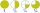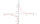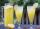# Fraction calculator

The calculator performs basic and advanced operations with fractions, expressions with fractions combined with integers, decimals, and mixed numbers. It also shows detailed step-by-step information about the fraction calculation procedure. Solve problems with two, three, or more fractions and numbers in one expression.

## Result:

### 81/6 / 17/8 = 196/45 = 4 16/45 ≅ 4.3555556

Spelled result in words is one hundred ninety-six forty-fifths (or four and sixteen forty-fifths).

### How do you solve fractions step by step?

1. Conversion a mixed number 8 1/6 to a improper fraction: 8 1/6 = 8 1/6 = 8 · 6 + 1/6 = 48 + 1/6 = 49/6

To find new numerator:
a) Multiply the whole number 8 by the denominator 6. Whole number 8 equally 8 * 6/6 = 48/6
b) Add the answer from previous step 48 to the numerator 1. New numerator is 48 + 1 = 49
c) Write a previous answer (new numerator 49) over the denominator 6.

Eight and one sixth is forty-nine sixths
2. Conversion a mixed number 1 7/8 to a improper fraction: 1 7/8 = 1 7/8 = 1 · 8 + 7/8 = 8 + 7/8 = 15/8

To find new numerator:
a) Multiply the whole number 1 by the denominator 8. Whole number 1 equally 1 * 8/8 = 8/8
b) Add the answer from previous step 8 to the numerator 7. New numerator is 8 + 7 = 15
c) Write a previous answer (new numerator 15) over the denominator 8.

One and seven eighths is fifteen eighths
3. Divide: 49/6 : 15/8 = 49/6 · 8/15 = 49 · 8/6 · 15 = 392/90 = 2 · 196 /2 · 45 = 196/45
Dividing two fractions is the same as multiplying the first fraction by the reciprocal value of the second fraction. The first sub-step is to find the reciprocal (reverse the numerator and denominator, reciprocal of 15/8 is 8/15) of the second fraction. Next, multiply the two numerators. Then, multiply the two denominators. In the next intermediate step, , cancel by a common factor of 2 gives 196/45.
In words - forty-nine sixths divided by fifteen eighths = one hundred ninety-six forty-fifths.

#### Rules for expressions with fractions:

Fractions - use the slash “/” between the numerator and denominator, i.e., for five-hundredths, enter 5/100. If you are using mixed numbers, be sure to leave a single space between the whole and fraction part.
The slash separates the numerator (number above a fraction line) and denominator (number below).

Mixed numerals (mixed fractions or mixed numbers) write as non-zero integer separated by one space and fraction i.e., 1 2/3 (having the same sign). An example of a negative mixed fraction: -5 1/2.
Because slash is both signs for fraction line and division, we recommended use colon (:) as the operator of division fractions i.e., 1/2 : 3.

Decimals (decimal numbers) enter with a decimal point . and they are automatically converted to fractions - i.e. 1.45.

The colon : and slash / is the symbol of division. Can be used to divide mixed numbers 1 2/3 : 4 3/8 or can be used for write complex fractions i.e. 1/2 : 1/3.
An asterisk * or × is the symbol for multiplication.
Plus + is addition, minus sign - is subtraction and ()[] is mathematical parentheses.
The exponentiation/power symbol is ^ - for example: (7/8-4/5)^2 = (7/8-4/5)2

#### Examples:

adding fractions: 2/4 + 3/4
subtracting fractions: 2/3 - 1/2
multiplying fractions: 7/8 * 3/9
dividing Fractions: 1/2 : 3/4
exponentiation of fraction: 3/5^3
fractional exponents: 16 ^ 1/2
adding fractions and mixed numbers: 8/5 + 6 2/7
dividing integer and fraction: 5 ÷ 1/2
complex fractions: 5/8 : 2 2/3
decimal to fraction: 0.625
Fraction to Decimal: 1/4
Fraction to Percent: 1/8 %
comparing fractions: 1/4 2/3
multiplying a fraction by a whole number: 6 * 3/4
square root of a fraction: sqrt(1/16)
reducing or simplifying the fraction (simplification) - dividing the numerator and denominator of a fraction by the same non-zero number - equivalent fraction: 4/22
expression with brackets: 1/3 * (1/2 - 3 3/8)
compound fraction: 3/4 of 5/7
fractions multiple: 2/3 of 3/5
divide to find the quotient: 3/5 ÷ 2/3

The calculator follows well-known rules for order of operations. The most common mnemonics for remembering this order of operations are:
PEMDAS - Parentheses, Exponents, Multiplication, Division, Addition, Subtraction.
BEDMAS - Brackets, Exponents, Division, Multiplication, Addition, Subtraction
BODMAS - Brackets, Of or Order, Division, Multiplication, Addition, Subtraction.
GEMDAS - Grouping Symbols - brackets (){}, Exponents, Multiplication, Division, Addition, Subtraction.
Be careful, always do multiplication and division before addition and subtraction. Some operators (+ and -) and (* and /) has the same priority and then must evaluate from left to right.

## Fractions in word problems:

• ChestnutsThree divisions of nature protectors participated in the collection of chestnut trees.1. the division harvested 1250 kg, the 2nd division by a fifth more than the 1st division and the 3rd division by a sixth more than the second division. How many tons of
• Fractions 4How many 2/3s are in 6?
• Ten fractionsWrite ten fractions between 1/3 and 2/3
• Product of two fractionsProduct of two fractions is 9 3/5 . If one of the fraction is 9 3/7. Find the other fraction.
• Equivalent fractions 2Write the equivalent multiplication expression. 2 1/6÷3/4
• Numbers divisionWith what number should be divided mixed number 2 3/4 to get 11/12?
• Two dividedTwo divided by nine-tenths.
• A quotientWhat is the quotient of 3/10 divided by 2/4 as a fraction?
• ReciprocalsWhich among the given reciprocal is correct a. 3/15x1/3= 1 b. 3/20x20/3=1 c. 7/14x7/7=1 d. 34/3x34/34=1
• MilimetersHow many millimeters are 1/4 meters?
• LemonadeHow many 1/4 cup servings are in 2 and 1/3 cups of lemonade?
• 4/5 of4/5 of a number is 276. what is 2/3 of the same number?
• The quotientThe quotient of g and 55 is the same as 279. What is g?